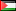##### Monte Carlo Simulation of Boltzmann Equation in Space Plasma at High Latitudes
Publication Type
Original research
Authors
• mad A. Barghouthi
• Naji A. Qatanani
• Fathi M. Allan
Fulltext

The Monte Carlo method was shown to be a very powerful technique in solving the Boltzmann equation by particle simulation. Its simple concept, straightforward algorithm, and its adaptability to include new features (such as, gravity, electric field, geomagnetic field, and different collision models) make it useful tool in space plasma physics, and a powerful test of results obtained with other mathematical methods. We have used Monte Carlo method to solve Boltzmann equation, which describes the motion of a minor ion in a background of ions under the effect of external forces and Coulomb collisions with background ions. We have computed the minor ion velocity distribution function, drift velocity, density, temperatures and heat fluxes. As an application, Monte Carlo simulation method has been adapted to determine the O + velocity distribution function, O + density, O + drift velocity, O + temperatures, and O + heat fluxes for Coulomb Milne problem.

Journal
Title
Monte Carlo Methods and Applications, Volume 9, Issue 3 (Sep 2003)
Publisher
--
Publisher Country
PalestinePublication Type
Both (Printed and Online)
Volume
--
Year
2003
Pages
--